# Trig Equations

A trig equation is similar to a regular equation in that we need to find a number to replace the letter that makes the equation correct. For example:We solve this to find that. We check it by replacingwith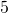:The solution tois.

A trig equation is different from a regular equation because there are generally many solutions, sometimes infinitely many.

The solution in the case of a simple trig equation refers to an angle. Trigonometry is useful to us in the context of something that changes but that repeats itself over time – such as the amount of moon we see each night which ranges from 0% to 100%. In applied trig equations the solution often refers to a time value – a trig equation is what we might use to calculate what day of the month we see a certain amount of the moon. We can apply trig to a context like this in the grade 12 precalculus course.

# Using the unit circle

Solve:This equation is asking for all the angles that give a sine value of 0.77, where those angles are between 0 and 360 degrees. That is, the first rotation of the unit circle.

We can find the answers by simply scrolling through angle on this applet until the green line (thecoordinate of the point on the circumference) measures 0.77. You can find two solutions:

By scrolling through angle we find thatand.

The solutions toareand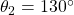. Or, since there is usually more than one solution we can write the solutions in a set:We don’t ordinarily have access to this applet and so we need an alternative approach using a scientific calculator only.

# Example 1

SolveFirst, we note that 0.31 is a positive number. Referring to the CAST diagram, we see that sine is positive in the first and second quadrant (marked ‘A’ and ‘S’ for ‘all’ and ‘sine’), that is, the upper half where thecoordinate is positive.Second, let’s find the reference angle – the angle that lies in the first quadrant. On the calculator, we findNow let’s draw a ‘bow-tie’ or a ‘stick-diagram’ on a circle, showing 18.1 degrees above and below the horizontal axis. Put a checkmark on the values in the first and second quadrant, because that is where sine is positive: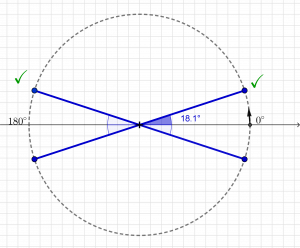We now calculate the number of degrees represented by the points at those two checkmarks. The calculation required in the second quadrant is.We have now found the two angles that have a sine value of 0.31.Finally, let’s check those two solutions:# Example 2

Solve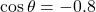First, we note that -0.8 is a negative number. Referring to the CAST diagram, we see that cosine is negative in the second and third quadrants, marked ‘S’ and ‘T’. In other words, where thecoordinate is negative.Second, let’s find the reference angle – the angle that lies in the first quadrant. We use positive 0.8 to find the first quadrant angle. There are other ways to proceed from here, be creative if you feel confident.Now let’s draw a ‘bow-tie’ or a ‘stick-diagram’ on a circle, showing 36.9 degrees above and below the horizontal axis. Put a checkmark on the values in the second and third quadrants, because that is where cosine is negative: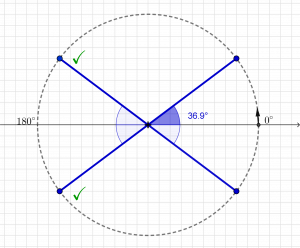We now calculate the number of degrees represented by the points at those two checkmarks. The calculations required areand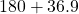.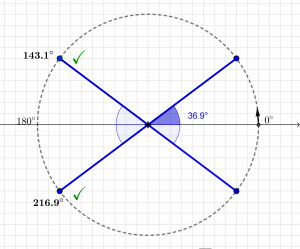We have now found the two angles that have a cosine value of -0.8.Finally, let’s check those two solutions:# Practice

practice simple trig equation applet

bowtie applet